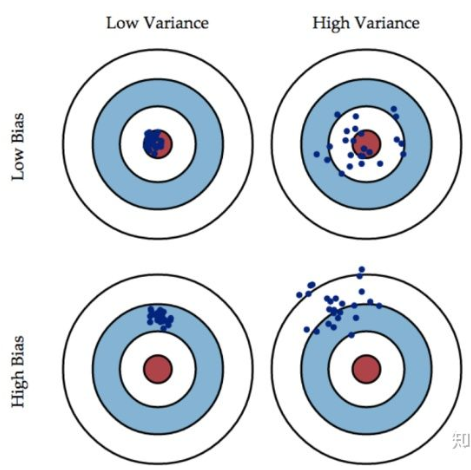# 单智能体强化学习算法

## TD3：Addressing Function Approximation Error in Actor-Critic Methods

Posted by MY on August 7, 2020

## 一、问题## 二、解法

### 2.1 Clipped Double Q-learning for Actor-Critic

$\begin{array}{l} y_{1}=r+\gamma Q_{\theta_{2}^{\prime}}\left(s^{\prime}, \pi_{\phi_{1}}\left(s^{\prime}\right)\right) \\ y_{2}=r+\gamma Q_{\theta_{1}^{\prime}}\left(s^{\prime}, \pi_{\phi_{2}}\left(s^{\prime}\right)\right) \end{array}$

$Q_{\theta_{1}}$和$Q_{\theta_{2}}$总会有高有低，高的值难免会有过估计的可能，所以文中在其基础上进行了稍微的改变，取两者之间的最小值：

$y=r+\gamma \min _{i=1,2} Q_{\theta_{i}^{\prime}}\left(s^{\prime}, \pi_{\phi_{1}}\left(s^{\prime}\right)\right)$

$Q_{\theta}(s, a)=r+\gamma \mathbb{E}\left[Q_{\theta}\left(s^{\prime}, a^{\prime}\right)\right]-\delta(s, a)$

$\begin{array}{l} Q_{\theta}\left(s_{t}, a_{t}\right)=r_{t}+\gamma \mathbb{E}\left[Q_{\theta}\left(s_{t+1}, a_{t+1}\right)\right]-\delta_{t} \\ =r_{t}+\gamma \mathbb{E}\left[r_{t+1}+\gamma \mathbb{E}\left[Q_{\theta}\left(s_{t+2}, a_{t+2}\right)-\delta_{t+1}\right]\right]-\delta_{t} \\ =\mathbb{E}_{s_{i} \sim p_{\pi}, a_{t} \sim \pi}\left[\sum_{i=t}^{T} \gamma^{i-t}\left(r_{i}-\delta_{i}\right)\right] \end{array}$

#### 2.2.1 Target Networks and Delayed Policy Updates

TD3中使用的第二个技巧就是对Policy进行延时更新，即使用target network。target network与critic并不同步更新，这样一来就可以减少之前我们提到的累计误差，从而降低方差。

#### 2.2.2 Target Policy Smoothing Regularization

$\begin{array}{l} y=r+\gamma Q_{\theta^{\prime}}\left(s^{\prime}, \pi_{\phi^{\prime}}\left(s^{\prime}\right)+\epsilon\right) \\ \epsilon \sim \operatorname{clip}(\mathcal{N}(0, \sigma),-c, c) \end{array}$

## 五、优点

• Clipped Double-Q Learning：使用两个Q函数进行学习，并在更新参数时使用其中最小的一个来避免value的过高估计。